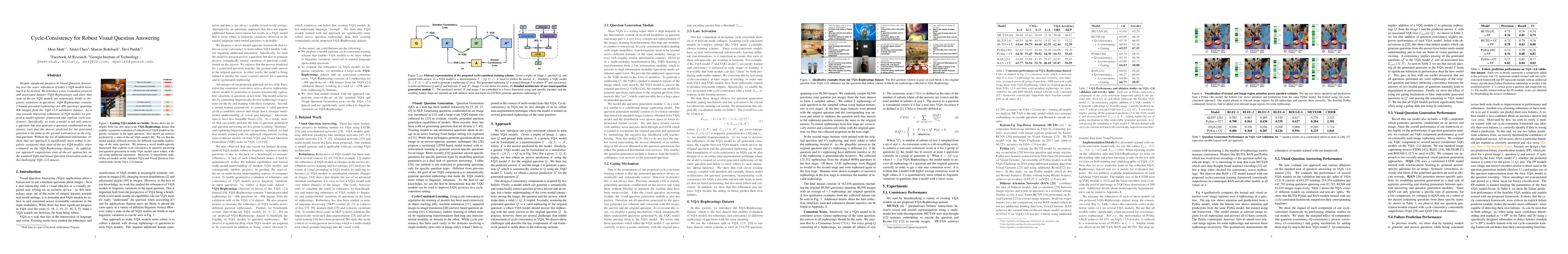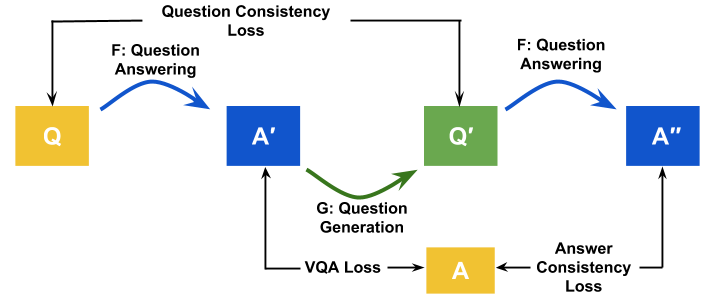## Paper

### Cycle Consistency for Robust Visual Question Answering

#### Meet Shah, Xinlei Chen, Marcus Rohrbach, Devi Parikh

##### CVPR 2019 (Oral)

Despite significant progress in Visual Question Answering over the years, robustness of today's VQA models leave much to be desired. We introduce a new evaluation protocol and associated dataset (VQA-Rephrasings) and show that state-of-the-art VQA models are notoriously brittle to linguistic variations in questions. VQA-Rephrasings contains 3 human-provided rephrasings for 40k questions spanning 40k images from the VQA v2.0 validation dataset.

As a step towards improving robustness of VQA models, we propose a model-agnostic framework that exploits cycle consistency. Specifically, we train a model to not only answer a question, but also generate a question conditioned on the answer, such that the answer predicted for the generated question is the same as the ground truth answer to the original question.

Without the use of additional annotations,we show that our approach is significantly more robust to linguistic variations than state-of-the-art VQA models, when evaluated on the VQA-Rephrasings dataset. In addition, our approach outperforms state-of-the-art approaches on the standard VQA and Visual Question Generation tasks on the challenging VQA v2.0 dataset.## ModelAbstract representation of the proposed cycle-consistent training scheme: Given a triplet of image $$I$$, question $$Q$$, and ground truth answer $$A$$, a Visual Question Answering (VQA) model is a transformation $$F:(Q,I)\mapsto A^\prime$$ used to predict the answer $$A^\prime$$. Similarly, a Visual Question Generation (VQG) model $$G:(A^\prime,I)\mapsto Q^\prime$$ is used to generate a rephrasing $$Q^\prime$$ of $$Q$$. The generated rephrasing $$Q^\prime$$ is passed through $$F$$ to obtain $$A^{\prime\prime}$$ and consistency is enforced between $$Q$$ and $$Q^\prime$$ and between $$A^{\prime}$$ and $$A^{\prime\prime}$$.

## Dataset

#### Dataset Format

VQA-Rephrasings contains 121,512 human-provided rephrasings for 40,504 original questions spanning 40,504 images from the VQA v2.0 validation dataset. The format of the questions is same as that of the VQA v2 dataset. Each question consists of one question from the VQA v2 validation split associated with 3 rephrasings. Each rephrasing has an additional field rephrasing_of which points to the question_id of the question it is a rephrasing of. More details about each field is provided in the schema table below. Consistency score as described in the paper can be found in the VQA-Eval repository.

Input Schema

{ question{ "question_id" : int, "image_id" : int, "rephrasring_of" : int, "coco_split" : str, "question" : str }

## People#### Meet Shah Facebook AI Research#### Xinlei Chen Facebook AI Research#### Marcus Rohrbach Facebook AI Research